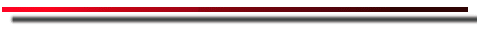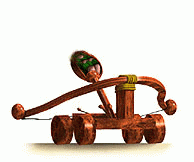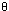# Catapult: Using Computers to do Solve Seemingly Simple ProblemsPurpose

The purpose of this lab is to show how computational science can be used to help solve a conceptually simple problem which involves a lot of calculations to solve. In this case, we consider the problem of projectile motion.

When projectile motion is taught in introductory physical science courses, air resistance is never really considered, even though we know that it can have a big effect on the motion of a projectile. After all, air resistance what prevents skydivers jumping out of airplanes from hitting the ground below at fatally high speed. The reason air resistance is often ignored is not because it is unimportant in the real world, but rather because of the large number of calculations necessary to compute an accurate trajectory when air resistance is included.

This lab is designed to show how a computer can solve this conceptually simple problem very easily, precisely because it involves a large number of repeated calculations (something computers were designed to do).

Words to Know the Meanings of before Continuing

launch angle, air resistance, range, launch velocity, terminal velocity
glossary of terms

Background Science

Projectile motion is a very fimilar kind of motion. Examples include a stone thrown into the air, a ball rolling off the edge of a table, a spacecraft circling the earth, and of course a shot from a catapult. In introductory physical science classes, we often model projectile motion by treating the horizontal and vertical motions separately.

Splitting up projectile motion into its horizontal (x) and vertical (y) components can normally be accomplished because gravity only acts in the vertical direction. If we ignore air resistance (or assume air resistance is negligible) so that gravity is the only force acting on the projectile, the equations of its motion are very easy to solve. For example, the equation for the range (horizontal distance) a projectile travels is simply:

R = vo2 sin(2)/g

where v0 is the launch speed,is the launch angle, and g is the gravitational acceleration. For example, if you throw a baseball at 25 meters/second (about 60 miles per hour), at an angle of 30 degrees, you can compute the range to be:

R = (25 m/s)2 sin(2*30o) / (9.8 m/s2) = 55 m

So you can throw a baseball about 55 meters (about helf the length of a football field). Keep in mind, it is not critical you memorize this equation, just notice how simple it is to use.

However, our simple assumption that gravity is the only force acting on the projectile is incorrect, at least in the real world. On earth, air resistance plays a significant role in the distance a projectile will travel. And to make matters worse, in general air resistance depends on the speed of the object, so the air resistance will decrease if the speed decreases and will increase if the speed increases. To solve the problem of the projectile with air resistance, you have to compute the speed of the projectile at many instants through its motion to make sure you compute the air resistance accurately for the entire flight.

In this simulation you will be shooting a payload to a specific point down range. At first you will looking at how this problem will be solved neglecting air resistance. You will see that this is not a very difficult problem to solve. Then you will add in air resistance to the problem, which increases the number of calculations greatly, and you'll see how inaccurate the simple equation above (which neglected air resistance) can be.

Using the Computer to Solve a Projectile Problem

First, follow this link to practice using a computer to compute the trajectory of a projectile, both including and neglecting air resistance. Once you are done practicing, you should attempt to solve this realistic projectile problem. It is an example of a problem that is easily done by a computer because of the computer's speed.

Conclusion

Keep in mind this problem would not be easily solvable without a computer, but becomes fairly trival with one. Also keep in mind that the problem could get even more complex. We haven't even considered
• The effects of wind on the projectile
• For extremely long projectile motions (on the order of several kilometers), you have to take into account the Coriolis effect when figuring out the flight path
• Depending on the shape of the object, if it tumbles in flight, its air resistance will change in flight.
The point is that real world projectile problems can become very complex and require a huge number of computations. However, they are tractable with computers. This is all because of the computer's main advantage over humans, it is very quick at making repeated calculations. This does not mean the computer is smarter than a human, it is simply faster. Remember that it was a human that told the computer what to do (this is called programming). The computer alone would not have known what to do to solve the problem. But once the computer has been programmed, it is able to a lot of calculations quickly to help us solve our projectile problem in a reasonable amount of time.

Go back to the SpECS Homepage

Educator's Notes/Notes For Those Wanting All The Details

Don't read this unless you want to know a lot more about how the Cannon Applet works.

The Applet uses an iterative routine to figure out the trajectory of a projectile. The routine is as follows:

1) Get the position (x, y) and velocity (vx, vy) of the projectile.

2) Compute the acceleration on the object in each direction using the equations:

 ax = Fx/m = -bvx2/m ay = Fy/m = (mg - bvy2)/m

where b is the coefficent of drag. In our applet, the coefficent of drag is computed on the fly, using the terminal velocity, vterm, set by the user. Since the terminal velocity is defined as the velocity where the gravitational force is exactly balanced by the air resistance, so that ay = 0 and thus:

ax = 0 = mg -bvterm2

therefore the drag coefficient is

b = mg/vterm2

NOTE: For simplicity, we assume a projectile mass of 5 kilos during the calculation. However, it really doesn't matter because we compute the acceleration only, so we divide drag coefficent by the mass during the computation, taking the effect of mass out of the equation.

3) Once we compute the force, we take a time step (dt) of 0.01 seconds (by default, this value can be changed by the user) and compute the distance the projectile travels (dx and dy) in that time using the equations:

 dx = vx(dt) + ax(dt)2 dy = vy(dt) + ay(dt)2

NOTE: These are differential equations and therefore there is no (1/2) in front of the acceleration component of these equations, you can view the equations as having the form (v + a(dt))*(dt), which is the true form of the equation before simplification.

4) The program now computes the new instantaneous velocity of the projectile using the equations:

 vx = dx/dt vy = dy/dt

5) Check to make sure we haven't hit the ground yet, if not, go back to step 1, otherwise print out the total range and stop computing.

Go back to the SpECS Homepage